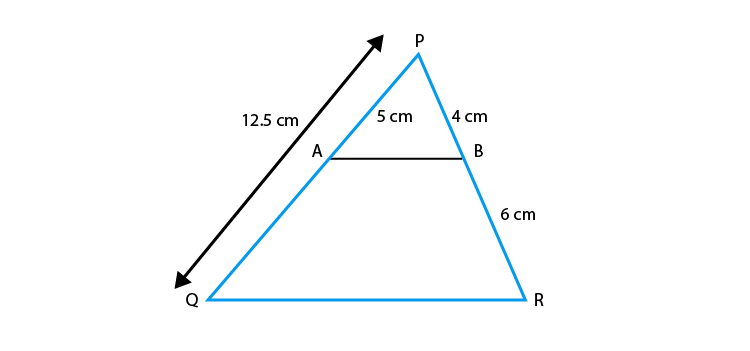# A and B are respectively the points on the sides PQ and PR of a ΔPQR such that PQ = 12.5 cm, PA = 5 cm, BR = 6 cm and PB = 4 cm. Is AB || QR? Give reason for your answer.

The given statement is True.

SolutionPQ = 12.5 cm

PA = 5 cm

BR = 6 cm

PB = 4 cm

So

QA = QP – PA = 12.5 – 5 = 7.5 cm

Hence

PA/AQ = 5/7.5 = 50/75 = 2/3 … (i)

PB/BR = 4/6 = 2/3 … (ii)

Form above quations (i) and (ii).

PA/AQ = PB/BR

We know that, if a line divides any two sides of a triangle in the same ratio, then the line is parallel to the third side.

Hence

AB || QR.(0)(0)